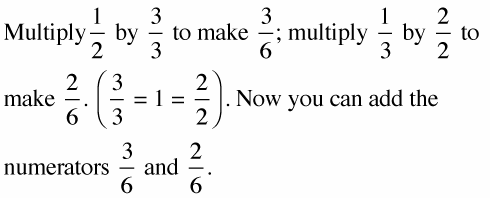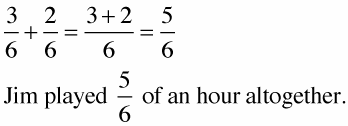##### Linear Algebra For Dummies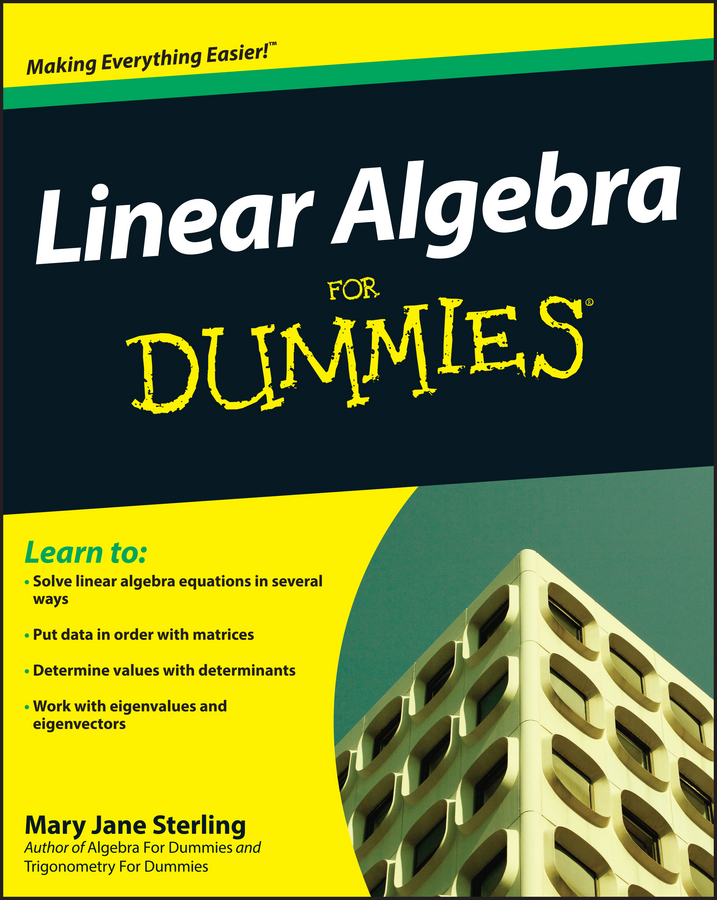Adding fractions is easy if the denominators are the same, but adding fractions with different denominators requires care. If you set up an algebraic equation to add fractions with different denominators, you need to find the lowest common denominator first.

The lowest common denominator of two or more numbers is the smallest whole number that is divisible by each.

After you convert the fractions so that they have common denominators, you just add the numerators, leaving the denominators alone. Then, if, necessary, you reduce the answer.

Example: Jim played for half an hour in yesterday’s soccer game and for 20 minutes in today’s game. How long did Jim play altogether?

1. Set up an equation.

If you begin with a word problem and not an equation, then you must “translate” the word problem into numbers.

Jim played for 1/2 hour one day and for 1/3 hour (20 minutes) another day, and you're trying to find out exactly how long he played for both days combined. The equation looks like this: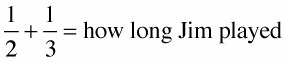2. Find the common denominator.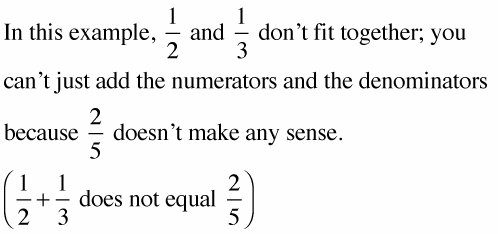You have to find a common denominator — that is, find the same number that each denominator will divide into evenly.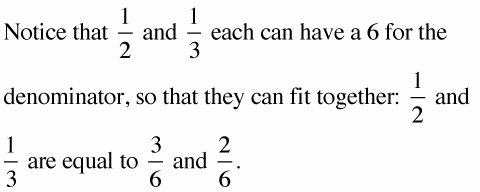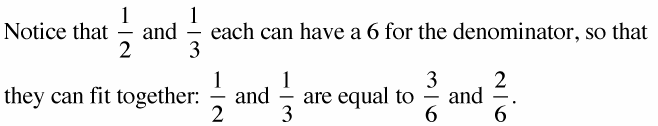So, you can fit the two fractions together by multiplying both the numerators and the denominators by the same number: